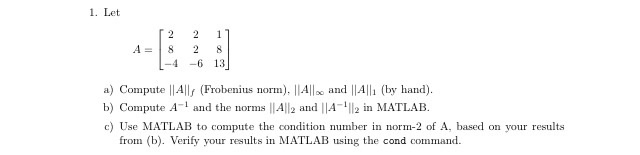Let  A = [2 8 -4  2 2 -6  1 8 13]   a) Compute ||A||_f (Frobenius norm), ||A||_infinity and ||A||_1 (by  hand).  b) Compute A^-1 and the norms ||A||_2 and ||A^-1||_2 in MATLAB.   c) Use MATLAB to compute the condition number in norm-2 of A, based on  your results from (b). Verify your results in MATLAB using the cond  command.• Posted: 24 days ago